#0
1
01. 云栖社区>
2. 数据分析>
3. 博客>
4. 正文

## 推荐 ：手把手教你用Python创建简单的神经网络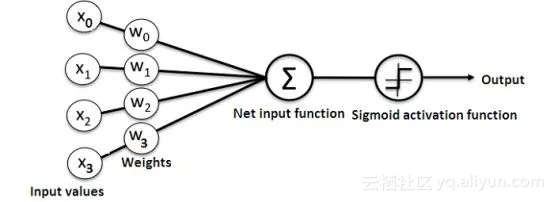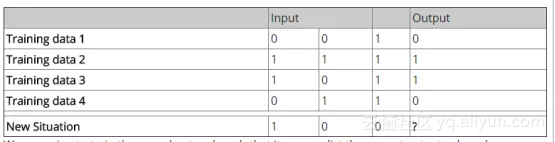numpy库提供了以下四种重要方法：

exp—用于生成自然指数

array—用于生成矩阵

dot—用于矩阵相乘

random—用于生成随机数。请注意，我们将生成随机数，以确保它们的有效分布。

1. 应用Sigmoid函数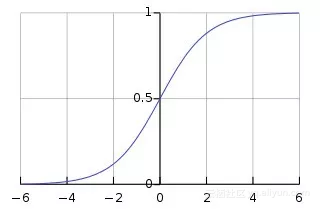1. 训练模型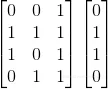import numpy as np

class NeuralNetwork():

def __init__(self):

# seeding for random number generation

np.random.seed(1)

#converting weights to a 3 by 1 matrix with values from -1 to 1 and mean of 0

self.synaptic_weights = 2 * np.random.random((3, 1)) - 1

def sigmoid(self, x):

#applying the sigmoid function

return 1 / (1 + np.exp(-x))

def sigmoid_derivative(self, x):

#computing derivative to the Sigmoid function

return x * (1 - x)

def train(self, training_inputs, training_outputs, training_iterations):

#training the model to make accurate predictions while adjusting weights continually

for iteration in range(training_iterations):

#siphon the training data via  the neuron

output = self.think(training_inputs)

#computing error rate for back-propagation

error = training_outputs - output

adjustments = np.dot(training_inputs.T, error * self.sigmoid_derivative(output))

def think(self, inputs):

#passing the inputs via the neuron to get output

#converting values to floats

inputs = inputs.astype(float)

output = self.sigmoid(np.dot(inputs, self.synaptic_weights))

return output

if __name__ == "__main__":

#initializing the neuron class

neural_network = NeuralNetwork()

print("Beginning Randomly Generated Weights: ")

print(neural_network.synaptic_weights)

#training data consisting of 4 examples--3 input values and 1 output

training_inputs = np.array([[0,0,1],

[1,1,1],

[1,0,1],

[0,1,1]])

training_outputs = np.array([[0,1,1,0]]).T

#training taking place

neural_network.train(training_inputs, training_outputs, 15000)

print("Ending Weights After Training: ")

print(neural_network.synaptic_weights)

user_input_one = str(input("User Input One: "))

user_input_two = str(input("User Input Two: "))

user_input_three = str(input("User Input Three: "))

print("Considering New Situation: ", user_input_one, user_input_two, user_input_three)

print("New Output data: ")

print(neural_network.think(np.array([user_input_one, user_input_two, user_input_three])))

print("Wow, we did it!")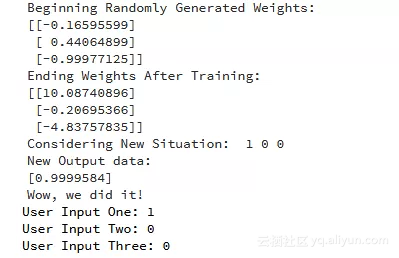ecshujufenxi”微信公众号

+ 关注